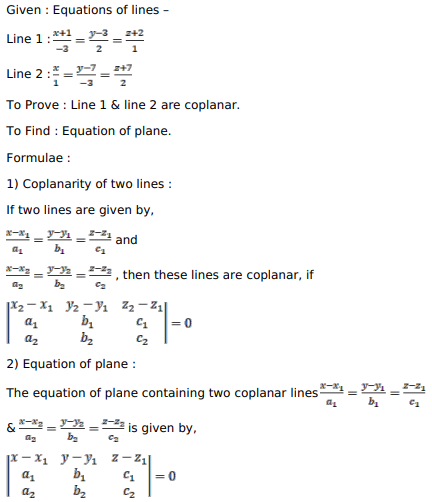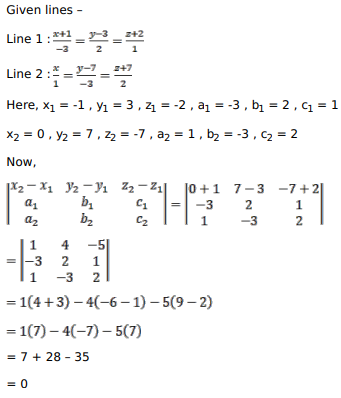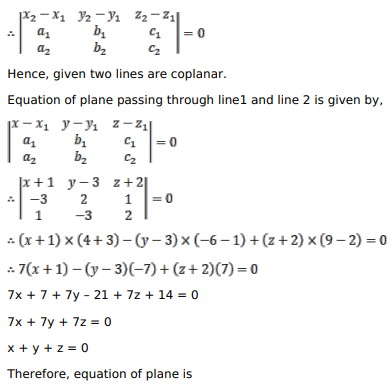# Solve this followingQuestion:

Show that the lines $\frac{x-1}{-3}=\frac{y-3}{2}=\frac{z-2}{1}$ and $\frac{x}{1}=\frac{y-7}{-3}=\frac{z+7}{2}$ are coplanar. Find the equation of the plane containing these lines.

Solution: